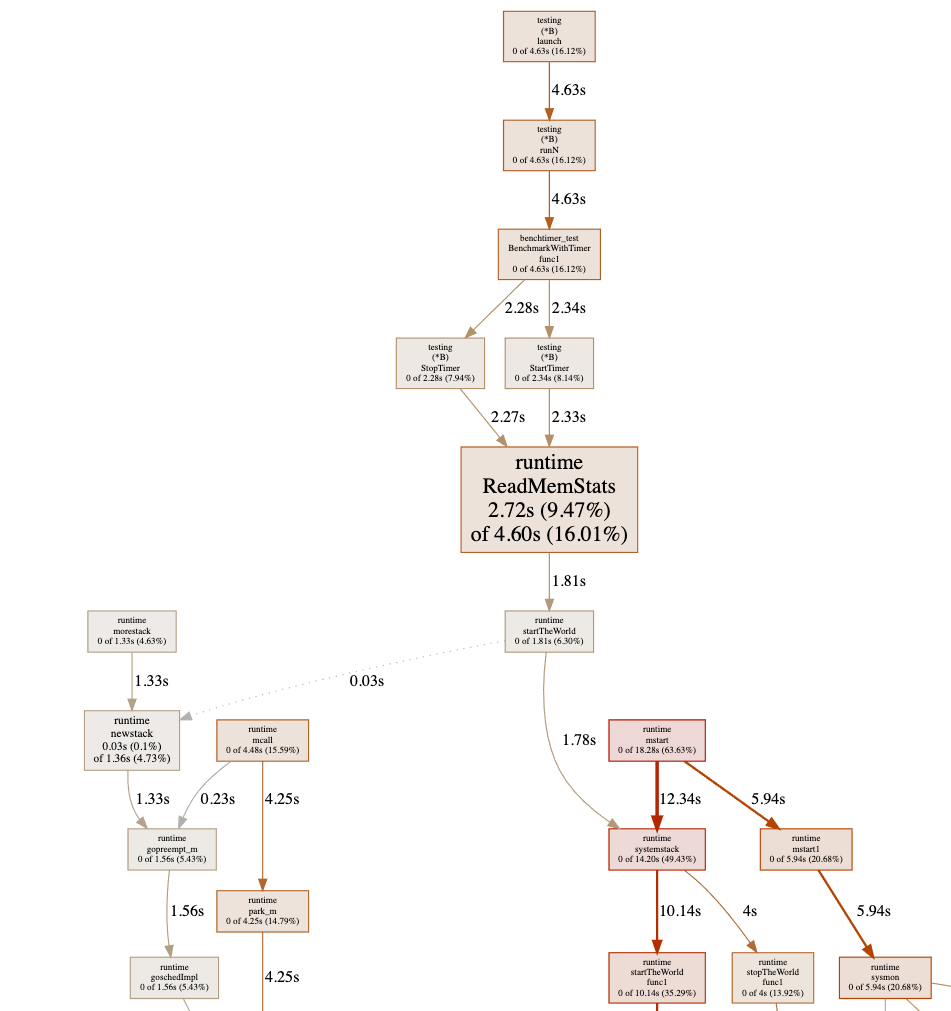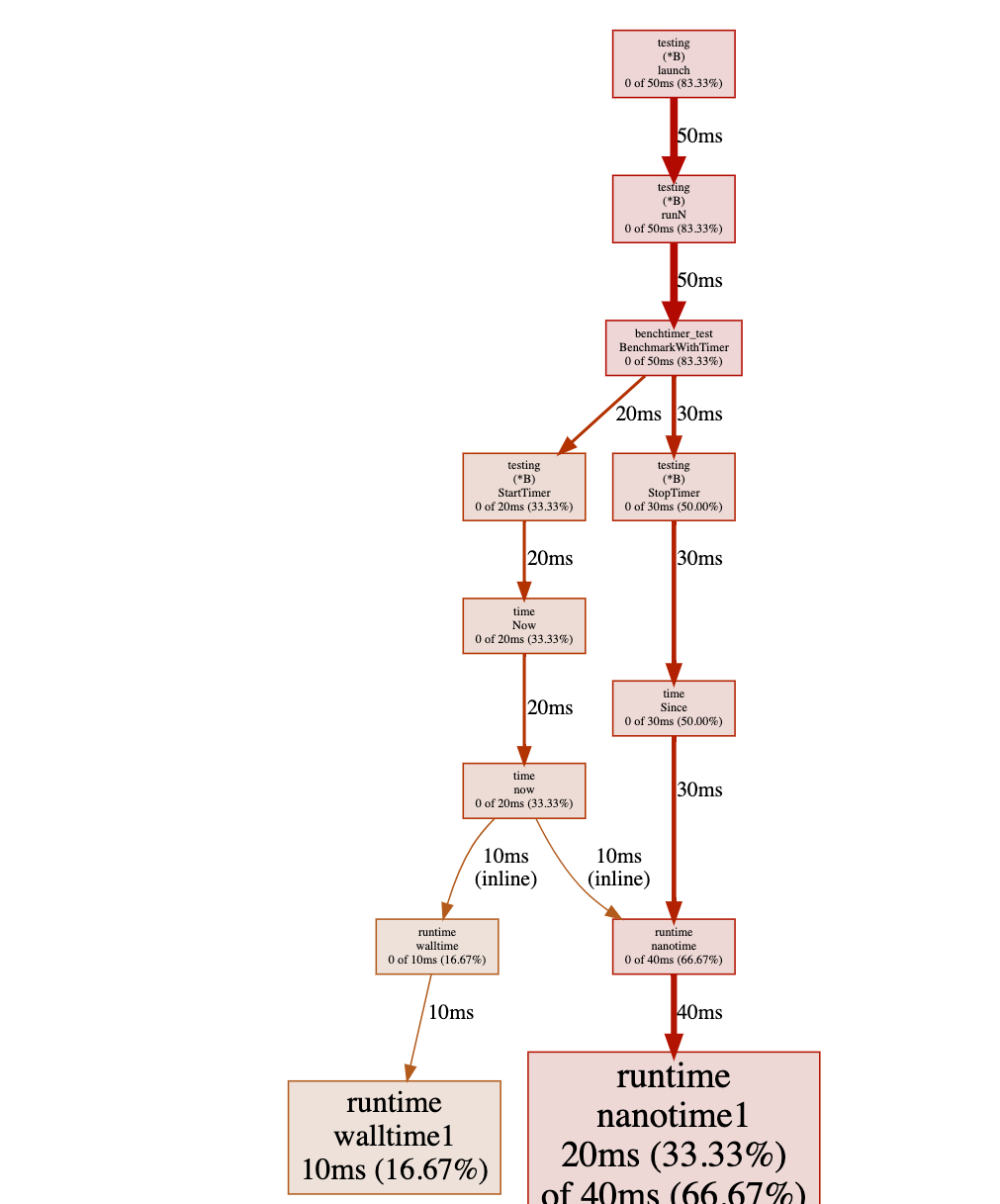┏━━━━━━━━━━━━━━━━━━━━━━━━┓

┗━━━━━━━━━━━━━━━━━━━━━━━━┛
(Possibly) Naïve thoughts regarding Go.

# Eliminating A Source of Measurement Errors in Benchmarks

| PV/UV:/ | PDF | #Benchmark #Error #TimeMeasurement

Author(s): Changkun Ou

About six months ago, I did a presentation1 that talks about how to conduct a reliable benchmark4 in Go. Recently, I submitted an issue #416412 to the Go project, which is also a subtle issue that you might need to address in some cases.

## Introduction

It is all about the following code snippet:

 1func BenchmarkAtomic(b *testing.B) {
2	var v int32
3	atomic.StoreInt32(&v, 0)
4	b.Run("with-timer", func(b *testing.B) {
5		for i := 0; i < b.N; i++ {
6			b.StopTimer()
7			// ... do extra stuff ...
8			b.StartTimer()
10		}
11	})
12	atomic.StoreInt32(&v, 0)
13	b.Run("w/o-timer", func(b *testing.B) {
14		for i := 0; i < b.N; i++ {
16		}
17	})
18}


On my target machine (CPU Quad-core Intel Core i7-7700 (-MT-MCP-) speed/max 1341/4200 MHz Kernel 5.4.0-42-generic x86_64), running this snippet with the following command:

go test -run=none -bench=Atomic -benchtime=1000x -count=20 | \
tee b.txt && benchstat b.txt


The result shows:

name                         time/op
Atomic/with-timer-8      32.6ns ± 7%
Atomic/w/o-timer-8       6.60ns ± 6%


Is it interesting to you? As you can see, the measurement without introducing StopTimer/StartTimer is 26ns faster than with the StopTimer/StartTimer pair. So, how is this happening?

To dig more reason behind it, let's modify the snippet a little bit:

 1func BenchmarkAtomic(b *testing.B) {
2	var v int32
3	var n = 1000000
4	for k := 1; k < n; k *= 10 {
5		b.Run(fmt.Sprintf("n-%d", k), func(b *testing.B) {
6			atomic.StoreInt32(&v, 0)
7			b.Run("with-timer", func(b *testing.B) {
8				for i := 0; i < b.N; i++ {
9					b.StopTimer()
10					b.StartTimer()
11					for j := 0; j < k; j++ {
13					}
14				}
15			})
16			atomic.StoreInt32(&v, 0)
17			b.Run("w/o-timer", func(b *testing.B) {
18				for i := 0; i < b.N; i++ {
19					for j := 0; j < k; j++ {
21					}
22				}
23			})
24		})
25	}
26}


This time, we use the k to increase the number of atomic operations in the bench loop, i.e.:

1for j := 0; j < k; j++ {
3}


Thus with higher k, the target code grows more costly. With similar command:

go test -run=none -bench=Atomic -benchtime=1000x -count=20 | \
tee b.txt && benchstat b.txt

name                          time/op
Atomic/n-1/with-timer-8       34.8ns ±12%
Atomic/n-1/w/o-timer-8        6.44ns ± 1%
Atomic/n-10/with-timer-8      74.3ns ± 5%
Atomic/n-10/w/o-timer-8       47.6ns ± 3%
Atomic/n-100/with-timer-8      488ns ± 7%
Atomic/n-100/w/o-timer-8       456ns ± 2%
Atomic/n-1000/with-timer-8    4.65µs ± 3%
Atomic/n-1000/w/o-timer-8     4.63µs ±12%
Atomic/n-10000/with-timer-8   45.4µs ± 4%
Atomic/n-10000/w/o-timer-8    43.5µs ± 1%
Atomic/n-100000/with-timer-8   444µs ± 1%
Atomic/n-100000/w/o-timer-8    432µs ± 0%


What's interesting in the modified benchmark result is by testing target code with a higher cost, the difference between with-timer and w/o-timer gets much closer. For instance, in the last pair of output when n=100000, the measured atomic operation only has (444µs-432µs)/100000 = 0.12 ns difference, which is pretty much accurate other than when n=1 the error is (34.8ns-6.44ns)/1 = 28.36 ns.

How is this happening? There are two ways to trace the problem down to the bare bones.

## Initial Investigation Using go tool pprof

As a standard procedure, let's benchmark the code that interrupts the timer and analysis the result using go tool pprof:

 1func BenchmarkWithTimer(b *testing.B) {
2	var v int32
3	for i := 0; i < b.N; i++ {
4		b.StopTimer()
5		b.StartTimer()
6		for j := 0; j < *k; j++ {
8		}
9	}
10}

go test -v -run=none -bench=WithTimer -benchtime=100000x -count=5 \
-cpuprofile cpu.pprof


Sadly, the graph shows a chunk of useless information where most of the costs shows as runtime.ReadMemStats:This is because of the StopTimer/StartTimer implementation in the testing package calls runtime.ReadMemStats:

 1package testing
2
3(...)
4
5func (b *B) StartTimer() {
6	if !b.timerOn {
8		b.startAllocs = memStats.Mallocs
9		b.startBytes = memStats.TotalAlloc
10		b.start = time.Now()
11		b.timerOn = true
12	}
13}
14
15func (b *B) StopTimer() {
16	if b.timerOn {
17		b.duration += time.Since(b.start)
19		b.netAllocs += memStats.Mallocs - b.startAllocs
20		b.netBytes += memStats.TotalAlloc - b.startBytes
21		b.timerOn = false
22	}
23}


As we know that runtime.ReadMemStats stops the world, and each call to it is very time-consuming. This is an known issue #208753 regarding runtime.ReadMemStats in benchmarking.

Since we do not care about memory allocation at the moment, to avoid this issue, let's just hacking the source code by just comment out the call to runtime.ReadMemStats:

 1package testing
2
3(...)
4
5func (b *B) StartTimer() {
6	if !b.timerOn {
7		// runtime.ReadMemStats(&memStats) // <- here
8		b.startAllocs = memStats.Mallocs
9		b.startBytes = memStats.TotalAlloc
10		b.start = time.Now()
11		b.timerOn = true
12	}
13}
14
15func (b *B) StopTimer() {
16	if b.timerOn {
17		b.duration += time.Since(b.start)
18		// runtime.ReadMemStats(&memStats) // <- here
19		b.netAllocs += memStats.Mallocs - b.startAllocs
20		b.netBytes += memStats.TotalAlloc - b.startBytes
21		b.timerOn = false
22	}
23}


And re-run the test again, then we have:Have you noticed where the problem is? Yes, there is a heavy cost in calling time.Now() in a tight loop (not really surprising because it is a system call).

## Further Verification Using C++

As you can see, the Go's pprof facility has its own problem while doing a benchmark, one can only edit the source code of Go to verify the source of the measurement error. Can we do something better than that?

Let's just write the initial benchmark in C++. This time, we go straightforward to the issue of now():

 1#include <iostream>
2#include <chrono>
3
4void empty() {}
5
6int main() {
7    int n = 1000000;
8    for (int j = 0; j < 10; j++) {
9        std::chrono::nanoseconds since(0);
10        for (int i = 0; i < n; i++) {
12            empty();
13            since += std::chrono::steady_clock::now() - start;
14        }
15        std::cout << "avg since: " << since.count() / n << "ns \n";
16    }
17}


compile it with:

clang++ -std=c++17 -O3 -pedantic -Wall main.cpp


In this code snippet, we are trying to measure the performance of an empty function. So, ideally, the output should be 0ns. However, there is still a cost in calling the empty function:

avg since: 17ns
avg since: 16ns
avg since: 16ns
avg since: 16ns
avg since: 16ns
avg since: 16ns
avg since: 16ns
avg since: 16ns
avg since: 16ns
avg since: 16ns


Furthermore, we could just simplify the code to the subtraction of two now() calls:

 1#include <iostream>
2#include <chrono>
3
4int main() {
5    int n = 1000000;
6    for (int j = 0; j < 10; j++) {
7        std::chrono::nanoseconds since(0);
8        for (int i = 0; i < n; i++) {
11        }
12        std::cout << "avg since: " << since.count() / n << "ns \n";
13    }
14}


and you could see that the output remains end in the cost of avg since: 16ns. This proves that there is an overhead of calling now() for benchmarking. Thus, in terms of benchmarking, the actual measured time of a target code equals to the execution time of target code plus the overhead of calling now():Assume the target code consumes in T ns, and the overhead of now() is t ns. Now, let's run the target code N times. The total measured time is T*N+t, then the average of a single iteration of the target code is T+t/N. Thus, the systematic measurement error becomes: t/N. Therefore with a higher N, you can get rid of the systematic error.

## The Solution

So, back to the original question, how can I get address the measurement error? A quick and dirty solution is just subtract the overhead of calling now():

 1#include <iostream>
2#include <chrono>
3
4void target() {}
5
6int main() {
7    int n = 1000000;
8    for (int j = 0; j < 10; j++) {
9        std::chrono::nanoseconds since(0);
10        for (int i = 0; i < n; i++) {
12            target();
13            since += std::chrono::steady_clock::now() - start;
14        }
15
18        since -= overhead * n;
19
20        std::cout << "avg since: " << since.count() / n << "ns \n";
21    }
22}


And in Go, you could do:

 1var v int32
2atomic.StoreInt32(&v, 0)
3r := testing.Benchmark(func(b *testing.B) {
4  for i := 0; i < b.N; i++ {
5    b.StopTimer()
6    // ... do extra stuff ...
7    b.StartTimer()
9  }
10})
11
12// do calibration that removes the overhead of calling time.Now().
13calibrate := func(d time.Duration, n int) time.Duration {
14  since := time.Duration(0)
15  for i := 0; i < n; i++ {
16    start := time.Now()
17    since += time.Since(start)
18  }
19  return (d - since) / time.Duration(n)
20}
21
22fmt.Printf("%v ns/op\n", calibrate(r.T, r.N))


As a take-away message, if you would like to write a micro-benchmark (whose runs in nanoseconds), and you have to interrupt the timer to clean up and reset some resources for some reason, then you must do a calibration on the measurement. If the Go's benchmark facility plans to fix #416412, then it is great; but if they don't, at least you are aware of this issue and know how to fix it now.

1. Changkun Ou. 2020. Conduct Reliable Benchmarking in Go. TalkGo Meetup. Virtual Event. March 26. https://golang.design/s/gobench

2. Changkun Ou. 2020. testing: inconsistent benchmark measurements when interrupts timer. The Go Project Issue Tracker. Sep 26. https://go.dev/issue/41641

3. Josh Bleecher Snyder. 2020. testing: consider calling ReadMemStats less during benchmarking. The Go Project Issue Tracker. Jul 1. https://go.dev/issue/20875

4. Beyer, D., Löwe, S. & Wendler, P. 2019. Reliable benchmarking: requirements and solutions. International Journal on Software Tools for Technology Transfer. Issue 21. https://doi.org/10.1007/s10009-017-0469-y